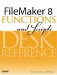# Average()

Category: Aggregate

 Syntax: Average ( field {; field...} )

Parameters:

fieldAny related field, repeating field, or set of non-repeating fields that represent a collection of numbers. Parameters in curly braces { } are optional and may be repeated as needed, separated by a semicolon.

Data type returned: Number

Description:

Returns a numeric value that is the arithmetic mean of all non-blank values in the set designated by the parameter list. The arithmetic mean of a set of numbers is the sum of the numbers divided by the size of the set. Blank values are not considered as part of the set.

When the parameter list consists of two or more repeating fields, Average() generates a repeating field in which the corresponding repetitions from the specified fields are averaged separately. So, if a field Repeater1 has two values, 16 and 20, and another field, Repeater2, has two values, 14 and 25, Average (Repeater1; Repeater2) would return a repeating field with values 15 and 22.5.

Examples:

Function

Results

Average (field1; field2; field3 )

Returns 2 when field1 = 1, field2 = 2, and field3 = 3.

Average ( repeatingField ) repeatingField; repeatingField; )

Returns 2 when repetition1 = 1, repetition2 = 2, and repetition3 = 3.

Average ( repeatingField;

Returns 2 when repetition1 = 1, repetition2 = 2, and repetition3 = 3.

Average ( Customer::InvoiceTotal)

Returns \$450 when a customer has three related invoice records with invoice totals of \$300, \$500, and \$550.FileMaker 8 Functions and Scripts Desk Reference
ISBN: 0789735113
EAN: 2147483647
Year: 2004
Pages: 352